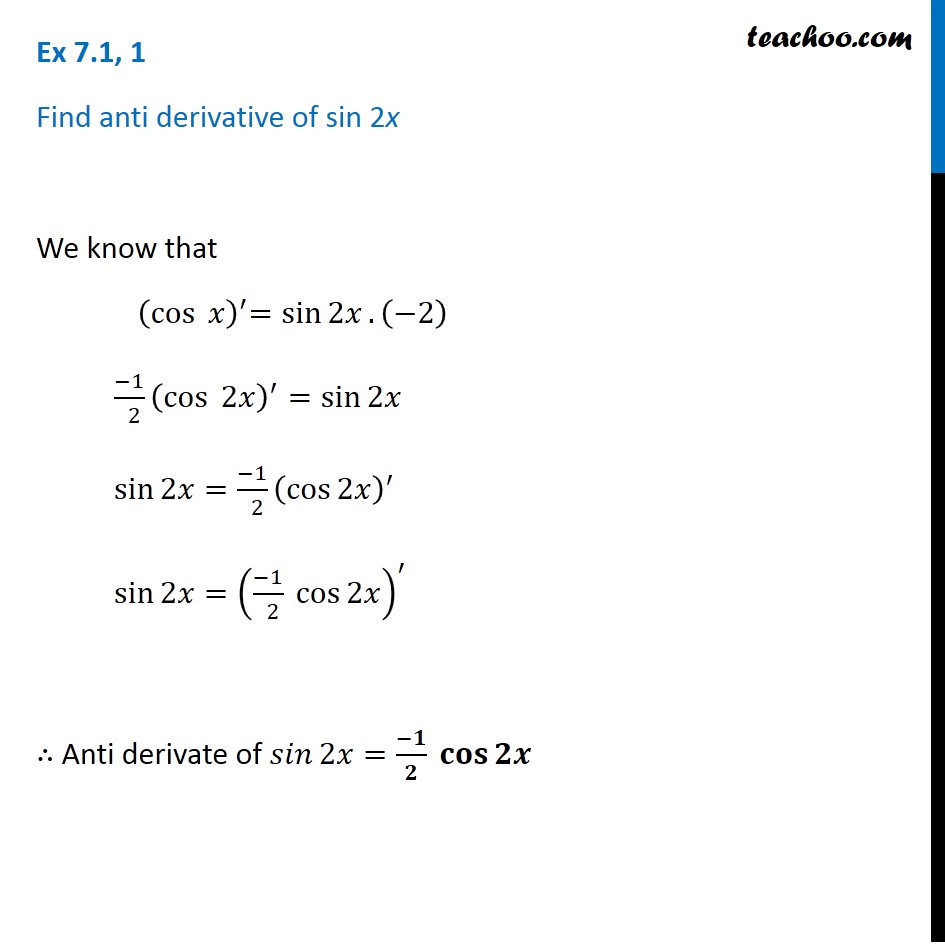1. Chapter 7 Class 12 Integrals (Term 2)
2. Serial order wise
3. Ex 7.1

Transcript

Ex 7.1, 1 Find anti derivative of sin 2x We know that 〖 (cos⁡〖 𝑥〗 )〗^′=sin⁡2𝑥. (−2) (−1)/( 2) (cos⁡〖 2𝑥〗 )^′=sin⁡2𝑥 sin⁡2𝑥=(−1)/( 2) (cos⁡2𝑥 )^′ sin⁡2𝑥=((−1)/( 2) cos⁡2𝑥 )^′ ∴ Anti derivate of 𝑠𝑖𝑛⁡2𝑥=(−𝟏)/𝟐 𝐜𝐨𝐬⁡𝟐𝒙

Ex 7.1